# Mixed Convection in Lid-driven Cavities Filled with a Nanofluid

Mixed Convection in Lid-driven Cavities Filled with a Nanofluid

I. ZeghbidR. Bessaïh

LEAP Laboratory, Department of Mechanical Engineering, Faculty of Sciences Technology, University of Frères Mentouri-Constantine, Route de Ain El. Bey, 25000 Constantine, Algeria

Corresponding Author Email:
leap.mechanical@gmail.com
Page:
77-84
|
DOI:
http://dx.doi.org/10.18280/ijht.330410
|
Accepted:
|
Published:
31 December 2015
| Citation

OPEN ACCESS

Abstract:

This present work concerns the study of laminar mixed convection in lid-driven cavities filled with Cu-water nanofluid. Each cavity is heated by two heat sources placed on vertical walls at a constant heat flux q''. The top wall moved with uniform velocity of U0. The top and bottom walls of the cavity are maintained at a local cold temperature TC, respectively. The continuity, Navier-Stokes, and energy equations are all solved by using the finite volume method. A computer program is developed and compared with the numerical results found in the literature. Results are presented in terms of streamlines, isotherms, vertical velocity profile, and average Nusselt numbers at a fixed Reynolds number (Re=10) and for Rayleigh numbers in the range (Ra=103-106), solid volume fractions of nanoparticles ($\phi$=0-0.20) and different aspect ratios (AR=0.5-4). It is found that the average Nusselt number increases with the increase of the Rayleigh number, solid volume fraction and aspect ratio of the cavity.

Keywords:

Mixed convection, Cavities, Nanofluid.

1. Introduction

Nanofluids are dispersions of nano-sized particles (having a diameter typically less than 100nm, called nanoparticles in a basic medium in order to improve certain properties. The most widely used fluids, such as water, oil or ethyleneglycol (EG) have only below thermal conductivity compared to that of crystalline solids. With nanofluids, the idea is to insert the nanoparticles in the base fluid in order to increase the effective thermal conductivity of the mixture. Choi  introduced the term nanofluid in 1985. Due to their excellent thermal performance, examples relate to heat exchangers, microchannels, cooling of electronic systems, vehicle cooling, nuclear reactors, buildings, grain storage, etc. A large number of studies on mixed convection with nanofluids have been done in recent years. Rahman et al. , Talebi et al. , and Pourmahmoud et al.  showed that the nanoparticles have a major influence on the flow and thermal fields. Mansour et al.  found that the type of nanofluids is a key factor for improving transfer, and the highest values ​​were observed when they used copper as nanoparticle. Salari et al.  presented the results of a numerical study of mixed convection within a fluid cavity filled with a nanofluid. The results show that the heat transfer increases with an increase in the Rayleigh, while it decreases with increasing volume fraction of the nanoparticle and Reynolds number. Abu-Nada et al.  numerically studied laminar mixed convection flow in a lid-driven inclined square enclosure filled with ananofluid.The results show that significant improvement of heat transfer could be obtained due to the presence of nanoparticles and the inclination of the enclosure. Mahmoudi  conducted a computer simulation on the natural convection  cooling  in  an

open cavity subjected to nanofluid (Cu-water). The results indicate that the average Nusselt number is an increasing function of the concentration of nanoparticles. Sebdania et al.  performed a numerical study of mixed convection in a square cavity filled with a nanofluid. The results show that when the heat source is in the middle, the effect of the addition of nanoparticles increases with increasing the Reynolds number, and that the heat transfer rate is decreased with increasing the solid volume fraction of nanoparticles. Alinia et al.  exhibited the thermal behavior of a nanofluid (SiO2-water) for different inclination angles of an inclined two-sided lid-driven cavity using two-phase mixture model. They found that the concentration (SiO2) nanoparticles remarkably increase the heat transfer rate. Akbari et al.  made a numerical simulation of mixed convection in horizontal and inclined tubes with uniform heat flux using a nanofluid. The results show that the concentration of Al2O3 is not significant effects on hydrodynamic parameters, and that the heat transfer coefficient is maximum when the angle of inclination is equal to 45°. Jmai et al.  and Mahmoudi et al.  examined the effects of parameters such as the Rayleigh number, the solid volume fraction of nanoparticles and the heat sources locations  on the natural convection flow in a partially heated cavity filled with different types of nanoparticles (Cu, Ag, Al2O3 and TiO2). They found that the heat transfer increases with the increasing of Rayleigh number and concentration of the nanoparticle, and the Cu-water nanofluid ensures a very high transfer versus nanofluids (water-Al2O3 and water-TiO2).

Arami et al.  dealt with the phenomenon of the sinusoidal heating side of a cavity filled with a nanofluid. The results show that the heat transfer rate increases with the decrease in Richardson and increasing the concentration of nanoparticles. Nasrin et al.  focused on the numerical modelling of laminar mixed convection flow in a wavy triangular cavity filled with a nanofluid. Mansour et al.  conducted an experimental study of mixed convection with a nanofluid in an inclined tube with uniform wall heat flux. Soleimani et al.  and Akbarinia et al.  numerically studied natural convection in a semi-annular cavity filled with a nanofluid. Shahi et al.  numerically investigated mixed convection cooling in a square cavity ventilated and partially heated from the below utilizing nanofluid.

The objective of this study is to investigate the laminar mixed convection in lid-driven cavities filled with Cu-water nanofluid and heated simultaneously by two heat sources. The effects of different aspect ratios on the flow and thermal fields, and on the average Nusselt number are studied for different Rayleigh numbers and solid volume fractions.

2. Geometry and Mathematical Model

The geometries considered in this study are illustrated in Figure 1.Each cavity filled with Cu-water nanofluid is heated by two heat sources placed on vertical walls at a constant heat flux q². The top wall moved with uniform velocity of U0. The top and bottom walls of the cavity are maintained at a local cold temperature TC, respectively. The vertical walls are adiabatic.

## 1a.png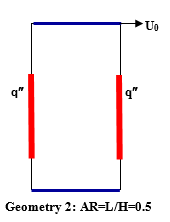## 1b.png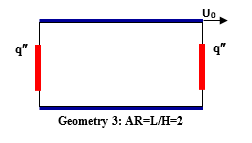## 1c.png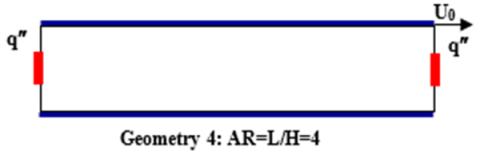Figure 1. Cavities for different aspect ratios filled with Cu-water nanofluid and containing two heat sources.

The thermo-physiques properties of the nanofluid are considered constants, and are assumed incompressible, Newtonian and laminar. Nanoparticles are supposed to have the shape and size uniform, and in a state of thermal equilibrium with the base fluid. Table 1 presents the thermo-physiques properties of (Cu) nanoparticles and the base fluid (pure water).

Table 1.Thermophysical properties of water and copper

 r (kg m-3) b (K-1) k  (W m-1 K-1) Cp (J kg-1K-1) Water Copper (Cu) 997.1 8933 21 × 10-5 1.67 × 10-5 0.613 401 4179 385

The equations of continuity, momentum, and energy with the above assumptions can be written as follows.

$\frac{\partial u}{\partial x}+\frac{\partial v}{\partial y}=0$     (1)

$u \frac{\partial u}{\partial x}+v \frac{\partial u}{\partial y}=\frac{1}{\rho_{n f}}\left[-\frac{\partial p}{\partial x}+\mu_{n f}\left(\frac{\partial^{2} u}{\partial x^{2}}+\frac{\partial^{2} u}{\partial y^{2}}\right)\right]$      (2)

$u \frac{\partial v}{\partial x}+v \frac{\partial v}{\partial y}=\frac{1}{\rho_{n f}}\left[-\frac{\partial p}{\partial y}+\mu_{n f}\left(\frac{\partial^{2} v}{\partial x^{2}}+\frac{\partial^{2} v}{\partial y^{2}}\right)\right]+(\rho \beta)_{n f} g\left(T-T_{C}\right)$       (3)

$u \frac{\partial T}{\partial x}+v \frac{\partial T}{\partial y}=\alpha_{n f}\left(\frac{\partial^{2} T}{\partial x^{2}}+\frac{\partial^{2} T}{\partial y^{2}}\right)$      (4)

The effective density of the nanofluid at reference temperature is:

rnf =  (1-f)rf + frp    (5)

The thermal expansion coefficient of nanofluid (rb) nf can be determined as follows:

(rb)nf= (1-f)(rb)f+ f(rb)p        (6)

The thermal diffusivity anf of the nanofluide can be expressed by

$\alpha_{n f}=\frac{k_{n f}}{\left(\rho c_{p}\right)_{n f}}$     (7)

The following relation (Brinkman ) determines the dynamic viscosity of the nanofluid:

$\mu_{n f}=\frac{\mu_{f}}{(1-\phi)^{2.5}}$     (8)

In this research, the thermal conductivity of nanofluids knf has been seen as being similar to other studies such as Rahman et al. , Mansour et al. , Salari et al. , and Abu-Nada and Shamkha  as follows.

$k_{n f}=\frac{k_{p}+2 k_{f}-2 \phi\left(k_{f}-k_{p}\right)}{k_{p}+2 k_{f}+\phi\left(k_{f}-k_{p}\right)} k_{f}$       (9)

Where kp is the thermal conductivity of dispersed nanoparticles, and kf is the thermal conductivity of pure fluid.

The dimensionless form of the governing equations using the following variables is:

X=x/L, Y=y/L, U=u/ U,V=v/ U0, P= p/ρnf U20, and q=T- TC /DT(=q² L/kf

$\mathrm{X}=\mathrm{x} / \mathrm{L}, \mathrm{Y}=\mathrm{y} / \mathrm{L}, \mathrm{U}=\mathrm{u} / \mathrm{U}_{0}, \mathrm{V}=\mathrm{v} / \mathrm{U}_{0}, \mathrm{P}=\mathrm{p} / \rho_{\mathrm{nf}} \mathrm{U}_{0}^{2},$ and $\theta=\mathrm{T}-\mathrm{T}_{\mathrm{C}} / \Delta \mathrm{T}\left(=\mathrm{q}^{\prime \prime} \mathrm{L} / \mathrm{k}_{\mathrm{f}}\right)$    (10)

The dimensionless governing equations are written as follows:

$\frac{\partial U}{\partial X}+\frac{\partial V}{\partial Y}=0$     (11)

$U \frac{\partial U}{\partial X}+V \frac{\partial U}{\partial Y}=-\frac{\partial P}{\partial X}+\frac{1}{\operatorname{Re}} \frac{\rho_{f}}{\rho_{n f}} \frac{1}{(1-\phi)^{2.5}}\left[\frac{\partial^{2} U}{\partial X^{2}}+\frac{\partial^{2} U}{\partial Y^{2}}\right]$   (12)

$U \frac{\partial V}{\partial X}+V \frac{\partial V}{\partial Y}=-\frac{\partial P}{\partial Y}+\frac{1}{\operatorname{Re}} \frac{\rho_{f}}{\rho_{n f}} \frac{1}{\left(1-\phi^{\prime}\right)^{2.5}}\left[\frac{\partial^{2} V}{\partial X^{2}}+\frac{\partial^{2} V}{\partial Y^{2}}\right] +\frac{(\rho \beta)_{n f}}{\rho_{n f} \beta_{f}} \frac{R a}{\operatorname{Pr} \operatorname{Re}^{2}} \theta$     (13)

$U \frac{\partial \theta}{\partial X}+V \frac{\partial \theta}{\partial Y}=\frac{\alpha_{n f}}{\alpha_{f}} \frac{1}{\operatorname{Re} \operatorname{Pr}}\left[\frac{\partial^{2} \theta}{\partial X^{2}}+\frac{\partial^{2} \theta}{\partial Y^{2}}\right]$      (14)

The boundary conditions are represented in dimensionless form, as follows:

At X=0:U= V=0, $\frac{\partial \theta}{\partial X}=0$ (left vertical wall)

At X=AR:U= V=0, $\frac{\partial \theta}{\partial X}=0$ (right vertical wall)

At Y=0: U= V=0, q=0 (horizontal wall)

At Y=1: U=1, V=0, q=0   (moving horizontal wall

Along the heat sources: U= V=0, $\frac{\partial \theta}{\partial X}=-\frac{k_{f}}{k_{n f}}$

The local Nusselt number on the surface of the heat source is defined as follows:

$\mathrm{Nu}=\frac{\mathrm{hL}}{\mathrm{k}_{\mathrm{f}}}$     (15)

where h is the heat transfer coefficient determined by

$\mathrm{h}=\frac{\mathrm{q}^{\prime \prime}}{\mathrm{T}_{\mathrm{s}}-\mathrm{T}_{\mathrm{C}}}$    (16)

By using the dimensionless variables mentioned above, the local Nusselt number becomes:

$\mathrm{Nu}_{\mathrm{s}}(Y)=\frac{1}{\theta_{\mathrm{s}}(Y)}$    (17)

Where qs is the dimensionless heat source temperature. Finally, the number of average Nusselt number along the heat source can be obtained by:

$\mathrm{Nu}_{\mathrm{m}}=\frac{1}{B} \int N u_{s}(Y) d Y$     (18)

Where, B(=b/L) is length of the heat source.

3. Numerical Method

The governing equations presented in Eqs. (11)-(14) along with the boundary conditions were solved by using our FORTRAN code, which using a control volume formulation (Patankar, ). The numerical procedure called SIMPLER (Patankar, ) was used to handle the pressure-velocity coupling. For treatment of the convection term in equations (13)-(15), power-law scheme was adopted. The convergence was obtained when the energy balance between the heat sources and the cold wall is less than a prescribed accuracy value, i.e., 0.2%.

A grid independent study was examined to ensure the accuracy and reliability of the numerical methods and to improve the accuracy of the simulation results. Six different mesh sizes were used: 42×42, 62×62, 82×82, 102×102, 122×82, and 122×122 nodes. For each mesh size, table 2 presents the average Nusselt (Num) and the dimensionless maximal temperature of the heat source qs,max for the MM case at Ra=105, Cu-water nanofluid, Re=10,  and f=0.10. The results show that a grid size of 122×122nodes satisfies the grid independence. This grid was, therefore, adopted for all numerical simulations.

Table 2. Grid Study results for a MM case (Ra=105, Cu-water, Re=10 and f = 0.10): Average Nusselt number along the heat source  and the maximum temperature of the heat source for different grids

 Grid 42×42 62×62 102×102 122×122 142×142 Num qs,max 6.9590 0.1880 6.8800 0.1860 6.8180 0.1840 6.8090 0.1840 6.8080 0.1840

To verify the accuracy of the present numerical study, the code was validated with the numerical results of Salari et al. . A comparison of the dimensionless vertical velocity V with X for Cu-water nanofluid, Reynolds number (Re=10), Rayleigh numbers(Ra=103, 104, 105 ), and two values of the solid volume fraction (f=0 and 0.20).  As shown in Figures 2a-b, it is clear that our results are in good agreement with the numerical results of Salari et al. .

## 2a.png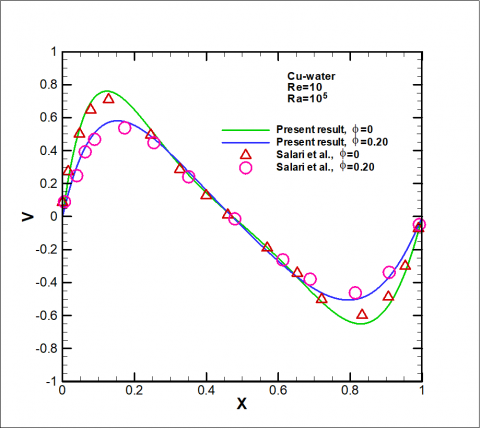(a)

Figure 2. (a) Validation of our code with the numerical results of Salari et al.  for  the dimensionless vertical velocity V with X in the central section of the square cavity filled with Cu-water nanofluid, at  Re=10, Ra = 105, f=0 and  f=0.20.

## 2b.png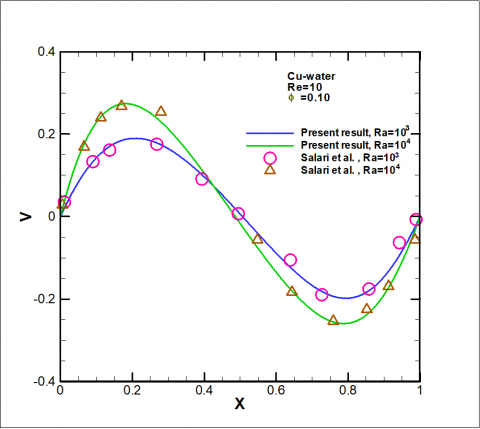(b)

Figure 2. (b) Validation of our code with the numerical results of Salari et al.  for the dimensionless vertical velocity V with X in the central section of the square cavity filled with Cu-water  nanofluid, at Re=10, f=0.10, Ra = 103 and 104.

4. Resultas and Discussion

To study the phenomenon of mixed convection in a rectangular cavity with two heat sources with Cu-water nanofluid, a series of calculations were conducted, in which the number of Rayleigh is a key factor. Simulations were conducted for Ra = 105 and 106, in order to see the effect of the Rayleigh number on the flow structure and heat transfer. We can be seen rotating inside the cavity through a recirculation cell near the movable wall (Figs.3a-b). The flow pattern consists of two cells of reverse rotation, the size of  recirculation zones  increases. This is due to the increase in the number of Rayleigh. This increase in the size of the area of circulation in the counterclockwise direction is used to produce heat to the main flow. Although,the recirculation zone is a region dead and isolated from the flow of the primary fluid, but in a counterclockwise direction in the area of recycling helps cool the cavity. It may be noted that temperature gradients are large enough close heat sources, against stratification observed in the velocity of the horizontal walls, brings significant thermal stratification the liquid to become colder, which considerably improves the cooling of the cavity.

## 3a.png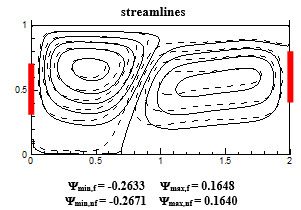## 3b.png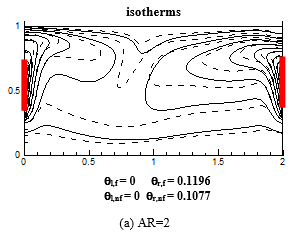## 3c.png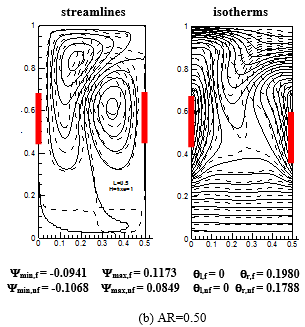Figure 3. Streamlines and isotherms for f=0(     ) and f=0.10 (    ) (Cu-water nanofluid) and two values of AR, at  Ra=106

Figures 4a-b show the streamlines and isotherms for f=0 and f=0.10 and two values of AR, at Ra=105. It was found that the presence of a heat source has a remarkable effect on the current function.

## 4a.png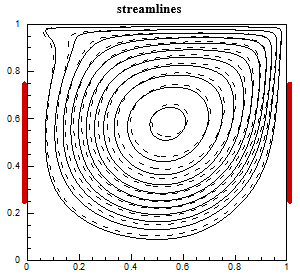## 4b.png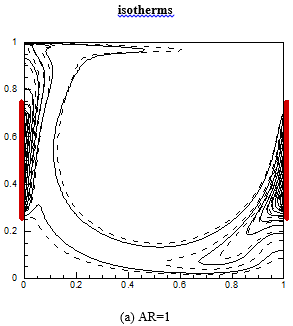## 4c.pngFigure 4. Streamlines and isotherms for f=0(     ) and f=0.10 (    ) (Cu-water nanofluid) and two values of AR, at Ra=105.

To examine the effect of the volume fraction of cu-water nanofluid on the variation of the heat transfer rate, simulations were performed to study the impact of the change in the Rayleigh number Ra = 103 and 106 on the average Nusselt number (Figure 5). Moreover, it was observed that the most important values ​​of the average Nusselt number were detected for an aspect ratio equal to AR = 4, the Num = 6.2 for Ra = 103 and  Num = 13 for Ra== 106, an increase of 52% was obtained. Also, the lowest value of the Nusselt number was obtained for an aspect ratio equal to AR = 0.5 and for a Rayleigh number equal to Ra = 103,  average Nusselt number equal to 4 and Ra = 106 the value of the average Nusselt number equal to 6, an increase of 33%.

Based on these results, we can appreciate the important role the aspect ratio on improving the heat transfer rate.

Therefore, one can conclude that the geometry with a high aspect ratio is the most favorable geometry that ensures a good transfer coefficient. (figure 5).

## 5a.png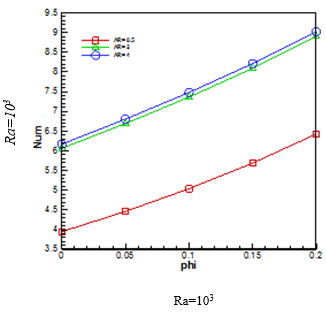## 5b.png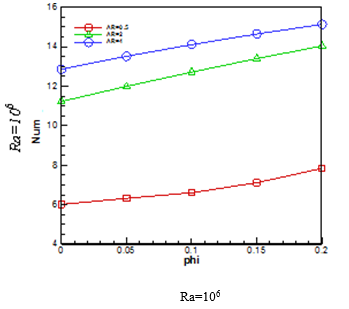Figure 5. Variation of the average Nusselt with f (phi) for different aspect ratios (AR = 0.5, 2 and 4), at Ra=103 and 106 and Re=10.

Figure 6a shows the variation of vertical velocity V with x as a function for different values of Ra, at different values of AR=0.5, 2 and Re=10. We can clearly see that for two aspect ratios AR = 0.5 and 2 and when we increase the Rayleigh number from Ra = 103 to 106, the vertical velocity experienced a greater increase compared to AR = 2, the value reaches 1 for Ra = 106, while for AR = 0.5 V is equal to 0.55 at the same Rayleigh number, an increase of 45%.Therefore, we can conclude that when we increase the aspect ratio, this directly affects the vertical velocity of the nanofluid in the cavity.

## 6a1.png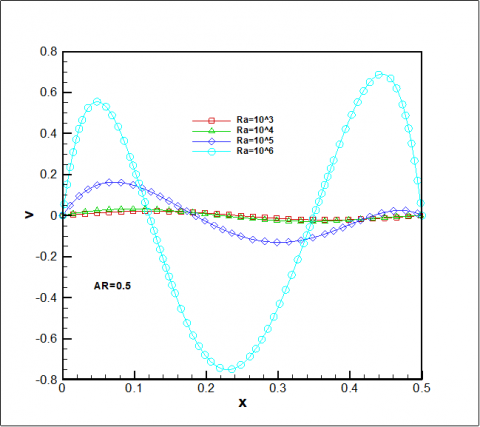## 6a2.pngFigure 6. (a) Variation of vertical velocity V with x for different values of Ra, at two values of AR=0.5, 2 and Re = 10.

Figure 6b illustrates the variation of vertical velocity V with x for different values of Ra, at two values of AR=0.5, 2 and Re = 10. it is found that AR = 05, and for a fluid without nanoparticles f= 0, the vertical velocity is 0.22, while when it increases the volume fraction of nanoparticles, it is noted that the fluid velocity decreases to 0.08, a decrease of  64%.

At AR = 2 and f=0, the vertical velocity is equal to 0.45, and becomes equal to 0.36 at f=0.20 , a decrease of 20% .Also, it is observed that the vertical velocity decreases significantly when  the aspect ratio AR is decreased from 2 to 0.5.Therefore, one can conclude that the volume concentration of nanoparticle is negatively effect on the vertical velocity of  the nanofluid.

## 6b1.png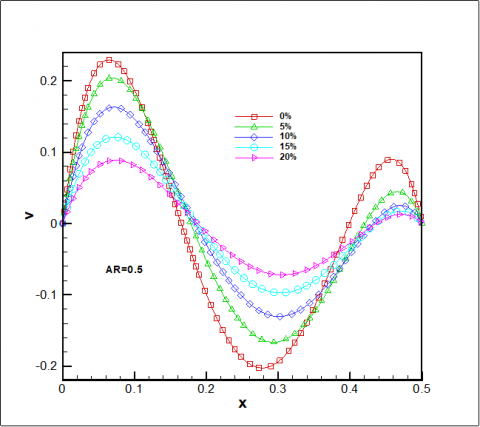## 6b2.png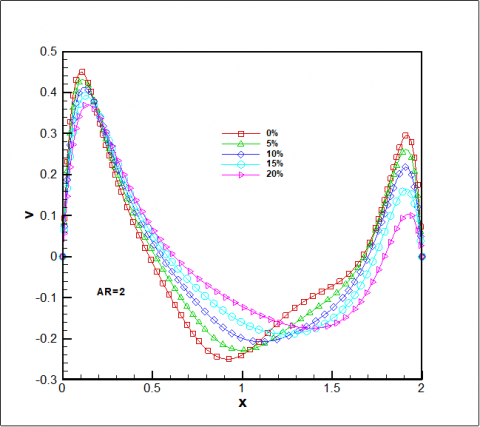Figure 6. (b) Variation of vertical velocity V with x for different values of  f at AR=0.5, 2 and Re = 10.

5. Conclusion

Laminar mixed convection in lid-driven cavities filled with a nanofluid has been studied. The finite volume method was used to solve the mathematical equations. A comparison with previously published work were in good agreement. The important concluding remarks are presented below:

•Increase the Rayleigh number, the average Nusselt number increases.

•The aspect ratio greatly affects the variation of the average Nusselt number.

•The geometry with a high aspect ratio is the most favorable geometry that ensures a good heat transfer rate.

•The increase of the aspect ratio affects the vertical velocity of the nanofluid in the cavity.

•The solid volume concentration has a negative  effect on the vertical velocity.

Nomenclature
 b length of the heat source, m d distance of the source with respect to the origin, m AR aspect ratio, L/H L width of the cavity, m g acceleration of gravity, m.s-2 k thermal conductivity, W/m.K H height of the cavity, m Num average Nusselt number along the heat source p pressure, Pa Pr Prandtl number, (nf / af) Ra Rayleigh number, (g bf H3DT/nf af) Re Reynolds number, (Re= rf U0 H/ mf) u, v horizontal and vertical velocity components, m.s-1 q² heat flux per unit area, W.m-2 T temperature, K Greek symbols f solid volume fraction ρ density, kg.m-3 ψ dimensionless stream function q dimensionless temperature ν kinematic viscosity, m2.s-1 m dynamic viscosity, kg. m-1.s-1 a thermal diffusivity, m2.s-1 Subscripts f fluid nf nanofluid p nanoparticle m average
References

1. U.S., Choi, “Enhancing thermal conductivity of fluids with nanoparticules”, ASME Fluids Eng. Div., 231, pp. 99-105, 1995.

2. M.M. Rahman, M.M. Billah, M. Hasanuzzaman, R. Saidur, and N.A. Rahim, “Heat transfer enhancement of nanofluids in a lid-driven square enclosure”, Numerical Heat Transfer, Part A, 62, pp. 973-991, 2012. DOI: 10.1080/10407782.2012.715983.

3. F. Talebi, A.H. Mahmoudi, and M. Shahi, “Numerical study of mixed convection flows in a square lid-driven cavity utilizing nanofluid”, International Communications in Heat and Mass Transfer, 37, pp. 79-90, 2010. DOI: 10.1016/j.icheatmasstransfer.2009.08.013.

4. N. Pourmahmoud, A. Ghafouri, and I. Mirzal, “Numerical study of mixed convection heat transfer in lid-driven cavity utilizing nanofluid: Effect of type and model”, Thermal Science, 53-53, pp. 973-991. 2013. DOI: 10.2298/TSCI120718053P.

5. M.A. Mansour, R.A. Mohamed, M.M. Abd-Elaziz, Sameh E. Ahmed, “Numerical simulation of mixed convection flows in a square lid-driven cavity partially heated from below using nanofluid”, International Communications in Heat and Mass Transfer, 37, pp.1504-1512, 2010. DOI: 10.1016/j.icheatmasstransfer.2010.09.004.

6. M. Salari, A.M. Tabar, and H.A. Danesh, “Mixed convection of nanofluid flows in a square lid-driven cavity heated partially from both the bottom and side walls”, Numerical Heat Transfer, Part A, 62, pp. 158-177, 2012. DOI: 10.1080/10407782.2012.691055.

7. E. Abu-Nada and A.J. Chamkha, “Mixed convection flow in a lid-driven inclined square enclosure filled with a nanofluid”, European Journal of Mechanics B/Fluids, 29, pp. 472-482, 2010. DOI: 10.1016/j.euromechflu.2010.06.008.

8. M. Mahmoudi, “Numerical simulation of free convection of nanofluid in a square cavity with an inside heater”, International Journal of Thermal Sciences, 50, pp. 2161-2175, 2011. DOI: 10.1016/j.ijthermalsci.2011.05.008.

9. S.M. Sebdania, M. Mahmoudi, and S.M. Hashemi, “Effect of nanofluid variable properties on mixed convection in a square cavity”, International Journal of Thermal Sciences, 52, pp.112-126. 2012. DOI: 10.1016/j.ijthermalsci.2011.09.003.

10. M. Alinia, D.D. Ganji, and M.Gorji-Bandpy, “Numerical study of mixed convection in an inclined two-sided lid-driven cavity filled with nanofluid using two-phase mixture model”, International Communications in Heat and Mass Transfer, 38, pp.1428-1435, 2011. DOI: 10.1016/j.icheatmasstransfer.2011.08.003.

11. M. Akbari, A. Behzadmehr, and F. Shahraki, “Fully developed mixed convection in horizontal and inclined tubes with uniform heat flux using nanofluid”, .International Journal of Heat and Fluid Flow, 29, pp. 545-556, 2008. DOI: 10.1016/j.ijheatfluidflow.2007.11.006.

12. R. Jmai, B. Ben-Beya, and T. Lili, “Heat transfer and fluid flow of nanofluid-filled enclosure with two partially heated sidewalls and different nanoparticules”, Superlattices and Microstructures, 53, pp. 130-154, 2013. DOI: 10.1016/j.spmi.2012.10.003.

13. M. Mahmoudi; S.M. Hashemi, “Numerical study of natural convection of a nanofluid in C-shaped enclosures”, International Journal of Thermal Sciences, 55, pp. 6-89, 2012. DOI: 10.1016/j.ijthermalsci.2012.01.002.

14. A.A. Arami, S.M. Sebdani, and M. Mahmoudi, “Numerical study of mixed convection flow in a lid-driven cavity with sinusoïdal heating on sidewalls using nanofluid”, Superlattices and Microstructures, 51, pp. 893-911, 2012. DOI: 10.1016/j.spmi.2012.02.015.

15. R. Nasrin, M.A. Alim, and A.J Chamkha, “Combined convection flow in triangular wavy chamber filled with water-Cu nanofluid: Effect of viscosity models”, International Communications in Heat and Mass Transfer, 39, pp. 1226-1236, 2012. DOI: 10.1016/j.icheatmasstransfer.2012.06.005.

16. R.B. Mansour, N. Galanis, and C.T. Nguyen, “Experimental study of mixed convection with water Al2o3 nanofluid in inclined tube with uniform wall heat flux”, International Journal of Thermal Sciences, 50, pp. 403-410. 2011. DOI: 10.1016/j.ijthermalsci.2010.03.016.

17. S. Soleimani, M.S. Oleslani, D.D. Ganji, and M.G. Banday, “Natural convection heat transfer in a nanofluid filled semi-annulus enclosure”, International Communications in Heat and Mass Transfer, 39, pp. 565-574, 2012. DOI: 10.1016/j.icheatmasstransfer.2012.01.016.

18. A. Akbarinia and A. Behzadmehr, “Numerical study of laminar mixed convection of a nanofluid in horizontal curved tubes”, Applied Thermal Engineering, 27, pp. 1327-1337, 2007. DOI: 10.1016/j.applthermaleng.2006.10.034.

19. M. Shahi, A.H. Mahmoudi, and F. Talebi, “Numerical study of mixed convection cooling in a square cavity ventilated and partially heated from the below utilizing nanofluid”, International Communications in Heat and Mass Transfer, 37, pp. 201-213, 2010. DOI: 10.1016/j.icheatmasstransfer.2009.10.002.

20. H.C. Brinkman, “The viscosity of concentrated suspensions and solution”, Journal of Chem. Phys. 20, pp. 571–581, 1952. DOI: 10.1063/1.1700493.

21. S.V. Patankar, Numerical Heat Transfer and Fluid Flow, Hemisphere, Washington, DC, 1980.